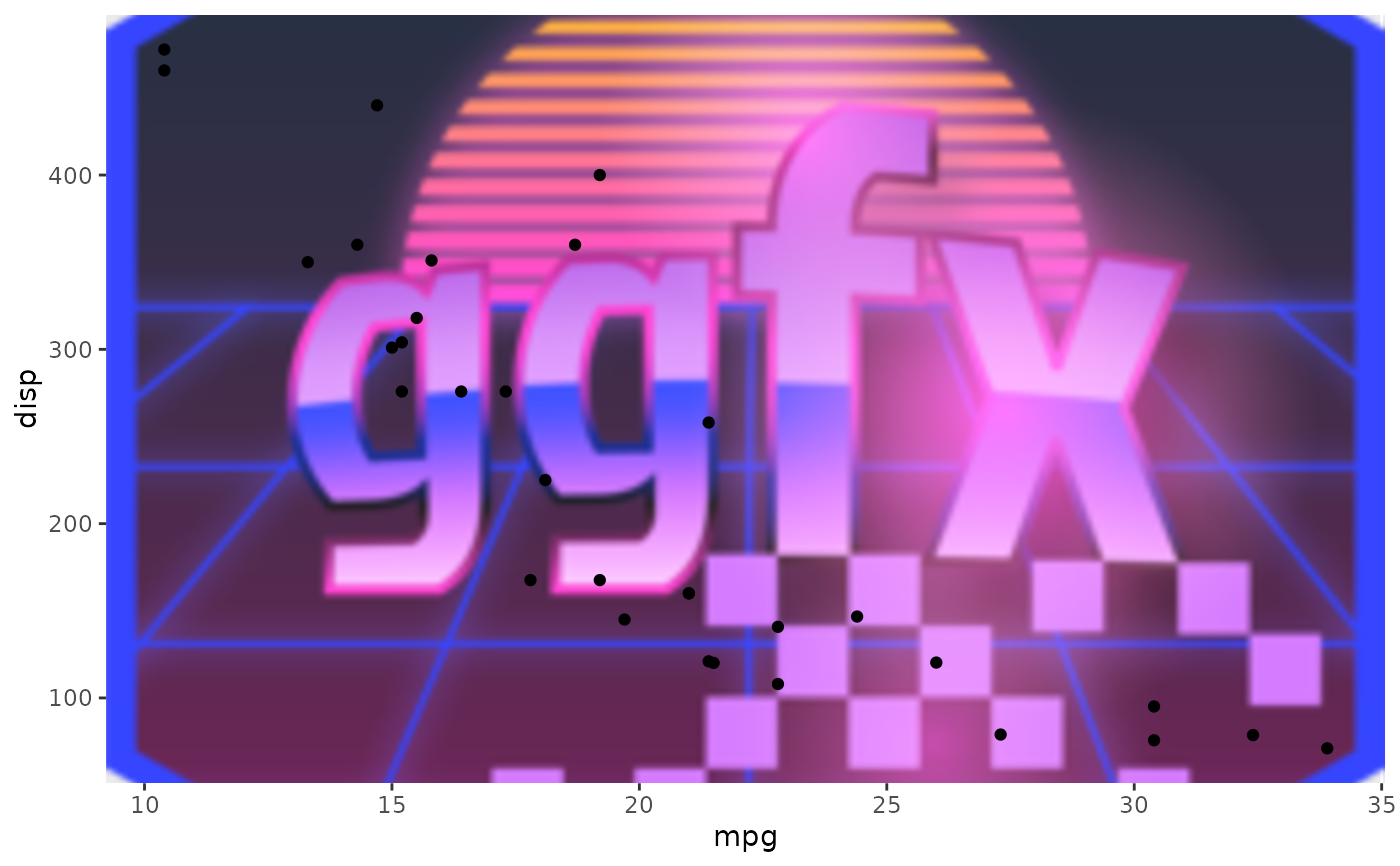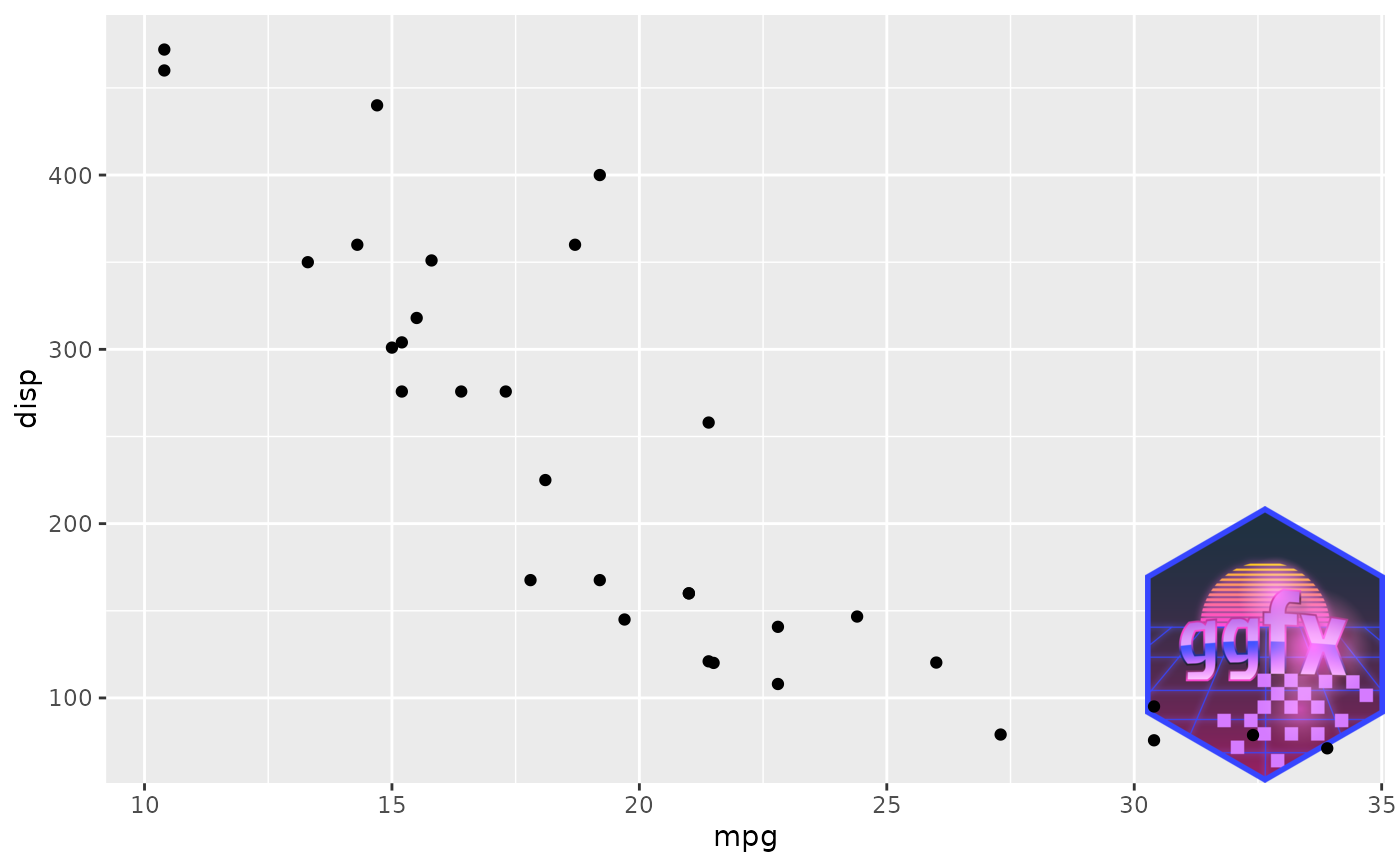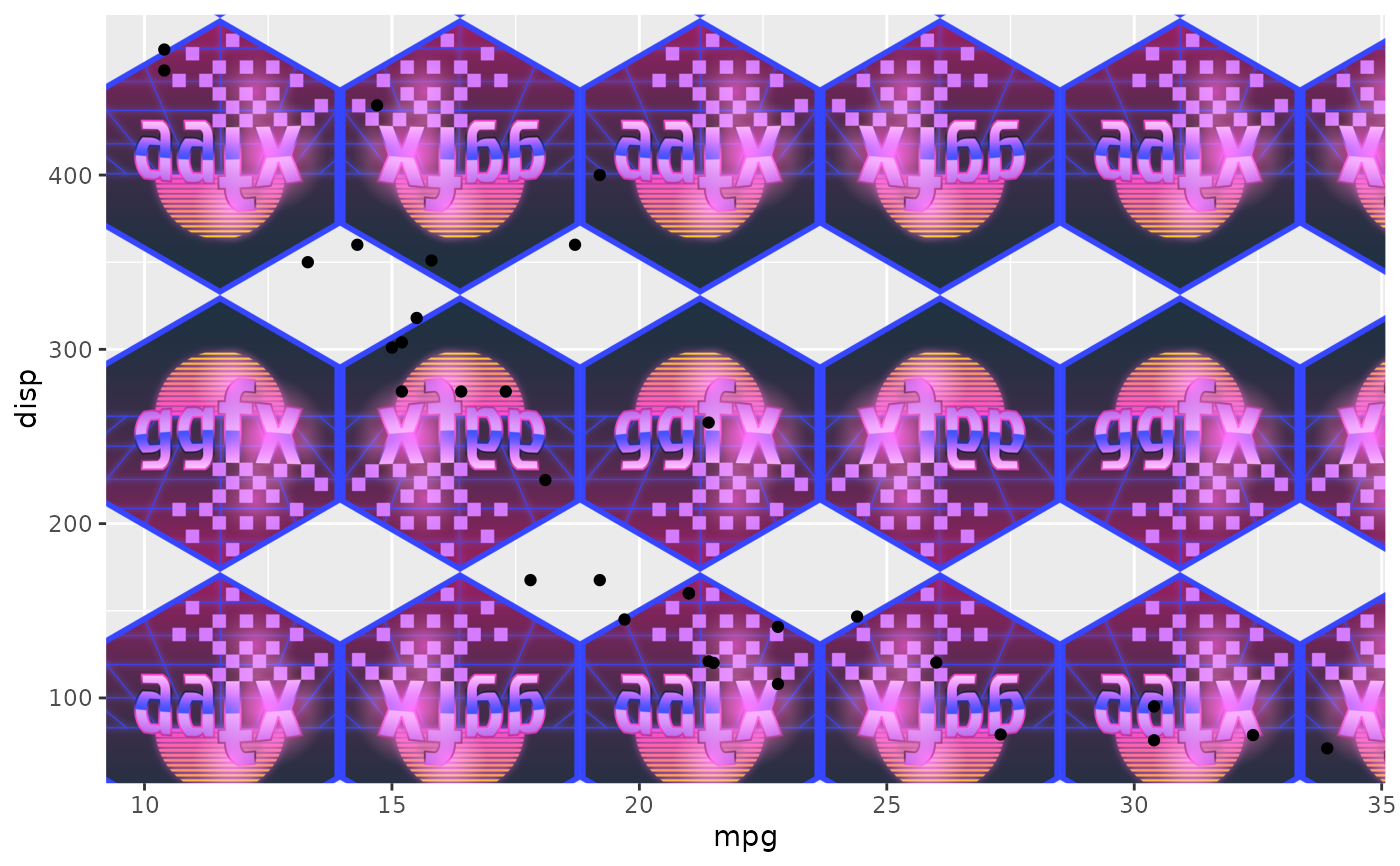When using raster objects directly you need to somehow define how it should be located in resized in the plot. These function can be used to inform the filter on how it should be used. They only work on raster type object, so cannot be used around functions or layer id's.

ras_fill(raster, align_to = "canvas")

ras_fit(raster, align_to = "canvas")

ras_stretch(raster, align_to = "canvas")

ras_place(raster, align_to = "canvas", anchor = "topleft", offset = c(0, 0))

ras_tile(
raster,
align_to = "canvas",
anchor = "topleft",
offset = c(0, 0),
flip = FALSE
)

## Arguments

raster

A raster or nativeRaster object or an object coercible to a raster object

align_to

Should the raster be positioned according to the canvas or the current viewport

anchor

Where should the raster be placed relative to the alignment area

offset

A unit or numeric vector giving an additional offset relative to the anchor. Positive values moves right/down and negative values move left/up

flip

Should every other repetition be flipped

## Value

The input with additional information attached

## Examples

library(ggplot2)
system.file('help', 'figures', 'logo.png', package = 'ggfx')
))

# Default is to fill the viewport area, preserving the aspect ratio of the
# raster
ggplot(mtcars) +
with_blend(
geom_point(aes(mpg, disp)),
logo
)# But you can change that with these functions:
ggplot(mtcars) +
with_blend(
geom_point(aes(mpg, disp)),
ras_place(logo, 'vp', 'bottomright')
)# Here we tile it with flipping, centering on the middle of the canvas
ggplot(mtcars) +
with_blend(
geom_point(aes(mpg, disp)),
ras_tile(logo, anchor = 'center', flip = TRUE)
)